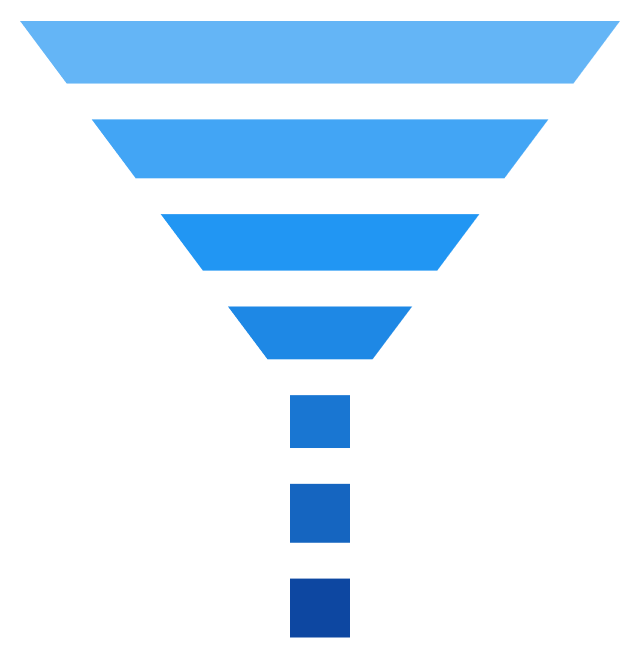# Funnel and Pyramid in WinUI Chart

25 May 20225 minutes to read

## Pyramid

`PyramidSeries` has the form of a triangle with lines dividing it into sections, and each section has a different width. Depending on the Y co-ordinates, this width indicates a level of hierarchy among other categories.

``<chart:PyramidSeries XBindingPath="Category" ItemsSource="{Binding Data}" YBindingPath="Value"/>``
``````PyramidSeries series = new PyramidSeries()
{

ItemsSource = new ViewModel().Data,

XBindingPath = "Category",

YBindingPath = "Value"

};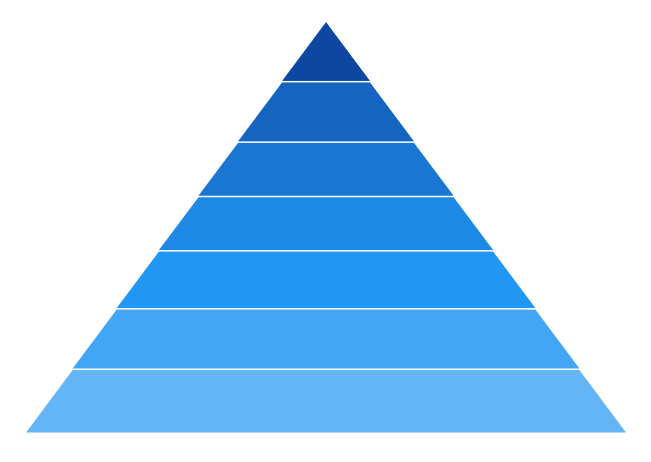The `PyramidMode` is used to define the rendering mode, such as `Surface` or `Linear` pyramid segments.

PyramidMode as Surface

``<chart:PyramidSeries XBindingPath="Category" PyramidMode="Surface" ItemsSource="{Binding Data}" YBindingPath="Value"/>``
``````PyramidSeries series = new PyramidSeries()
{

ItemsSource = new ViewModel().Data,

XBindingPath = "Category",

YBindingPath = "Value",

PyramidMode = ChartPyramidMode.Surface

};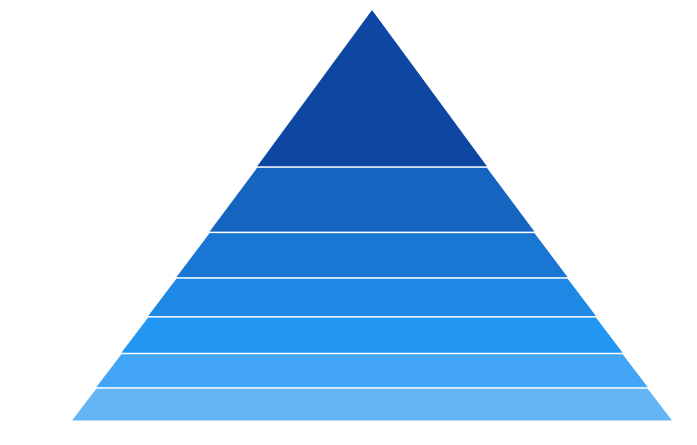PyramidMode as Linear

``<chart:PyramidSeries XBindingPath="Category" PyramidMode="Linear" ItemsSource="{Binding Data}" YBindingPath="Value"/>``
``````PyramidSeries series = new PyramidSeries()
{

ItemsSource = new ViewModel().Data,

XBindingPath = "Category",

YBindingPath = "Value",

PyramidMode = ChartPyramidMode.Linear

};## Funnel

`FunnelSeries` is similar to `PyramidSeries`, displays data in a funnel shape that equals to 100% when totaled. It is a single series, representing data as portions of 100%, and does not use any axes.

The following code example shows how to use the funnel series:

``<chart:FunnelSeries XBindingPath="Category" ItemsSource="{Binding Data}" YBindingPath="Value"/>``
``````FunnelSeries series = new FunnelSeries()
{

ItemsSource = new ViewModel().Data,

XBindingPath = "Category",

YBindingPath = "Value",

};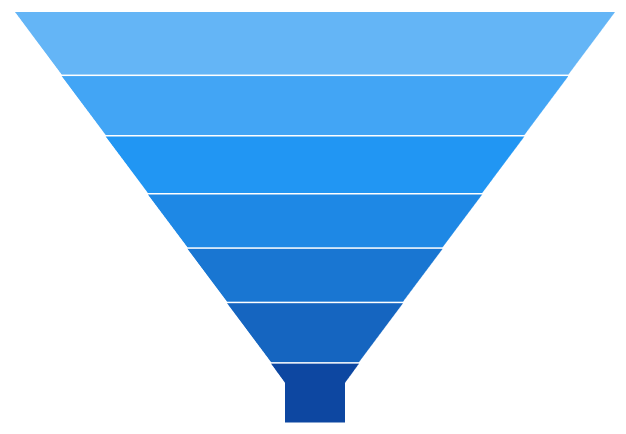### Funnel mode

The `FunnelMode` defines a rendering mode for the funnel series that specifies where to bind your values (to height or width). The following example demonstrates `ValueIsHeight` and `ValueIsWidth` funnel modes:

ValueIsHeight

``<chart:FunnelSeries XBindingPath="Category" ItemsSource="{Binding Data}" FunnelMode="ValueIsHeight" YBindingPath="Value"/>``
``````FunnelSeries series = new FunnelSeries()
{

ItemsSource = new ViewModel().Data,

XBindingPath = "Category",

YBindingPath = "Value",

FunnelMode = ChartFunnelMode.ValueIsHeight

};ValueIsWidth

``<chart:FunnelSeries XBindingPath="Category" ItemsSource="{Binding Data}" FunnelMode="ValueIsWidth" YBindingPath="Value"/>``
``````FunnelSeries series = new FunnelSeries()
{

ItemsSource = new ViewModel().Data,

XBindingPath = "Category",

YBindingPath = "Value",

FunnelMode = ChartFunnelMode.ValueIsWidth

};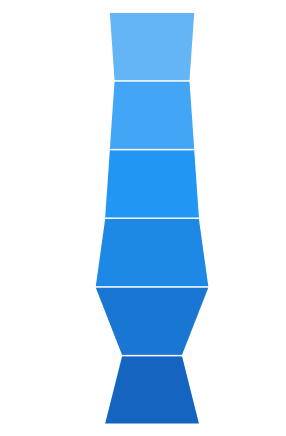### Explode segments

The following properties are used to explode the individual segments in Funnel and Pyramid.

Explode Offset

``````<chart:FunnelSeries XBindingPath="Category" ItemsSource="{Binding Data}"

ExplodeIndex="4"  ExplodeOffset="70" YBindingPath="Value">

</chart:FunnelSeries>``````
``````FunnelSeries series = new FunnelSeries()
{

ItemsSource = new ViewModel().Data,

XBindingPath = "Category",

YBindingPath = "Value",

ExplodeIndex = 4,

ExplodeOffset = 70

};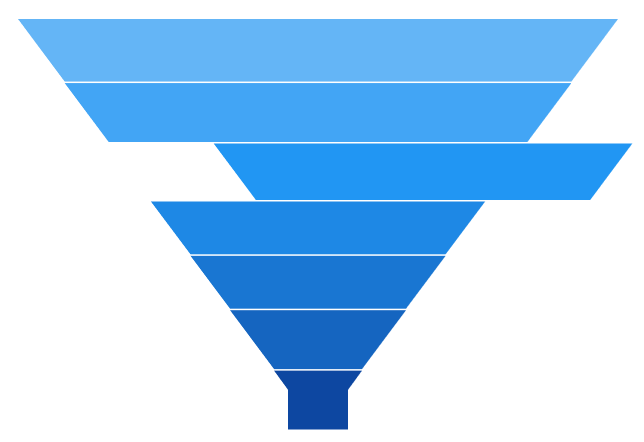Gap Ratio

The gap between each segment is calculated using the `GapRatio` property, as shown in the following code example.

``````<chart:FunnelSeries XBindingPath="Category" ItemsSource="{Binding Data}"

GapRatio="0.5" YBindingPath="Value">

</chart:FunnelSeries>``````
``````FunnelSeries series = new FunnelSeries()
{

ItemsSource = new ViewModel().Data,

XBindingPath = "Category",

YBindingPath = "Value",

GapRatio = 0.5

};